Home / Blog / Data Science / Box Plot

# Box Plot

• July 08, 2023
• 8900
• 5### Meet the Author : Mr. Bharani Kumar

Bharani Kumar Depuru is a well known IT personality from Hyderabad. He is the Founder and Director of Innodatatics Pvt Ltd and 360DigiTMG. Bharani Kumar is an IIT and ISB alumni with more than 17 years of experience, he held prominent positions in the IT elites like HSBC, ITC Infotech, Infosys, and Deloitte. He is a prevalent IT consultant specializing in Industrial Revolution 4.0 implementation, Data Analytics practice setup, Artificial Intelligence, Big Data Analytics, Industrial IoT, Business Intelligence and Business Management. Bharani Kumar is also the chief trainer at 360DigiTMG with more than Ten years of experience and has been making the IT transition journey easy for his students. 360DigiTMG is at the forefront of delivering quality education, thereby bridging the gap between academia and industry.

## What is Box Plot?

Yesterday, I unintentionally ran upon a former classmate in a neighbouring club. We had a lot to catch up on as we hadn't seen one other in a long time. Our conversation finally moved to the future.

'Friend':
"Hey do you remember I always wished to pursue higher studies. I think this is the right time. I am planning to pursue my Masters - MBA (Master of Business Administration)"
'Me':
"Oh wow!!! Congratulations, so when are you starting and which university have you joined?"
'Friend':
" I have not yet finalized any university. I am willing to join any university, though."
'Me':
"What Universities did you shortlist?"

'Friend':
" I have selected a few. I am finding it difficult to choose. Can you help me decide?"

'Me':
" Oh sure! Share the information that you have collected!"
'Friend':
" Certainly!!"Yesterday, I unintentionally ran upon a former classmate in a neighbouring club. We had a lot to catch up on as we hadn't seen one other in a long time. Our conversation finally moved to the future.

I did my part of the research and collected a few more details about those Universities like:

• What sort of examination does one need to clear to apply?
• What are the minimum marks that one would need to score to secure admission in a University?
• What is the acceptance criteria of the University?
• What is the ratio of students to faculty?
• Are there any scholarship programs? If so, what is the maximum scholarship allowed?
• What would be the minimum expenses?
• What would be the salary package for graduates of the University? etc.

One of the university webpages included a message that drew my eye. "Students at the University earn an average of \$132000 annually,"

I had gathered and organised the data necessary to begin my research, and I also had some intriguing thoughts to share with my buddy.

First Moment Business Decision or Measure of Central Tendency

• Mean - Average of all the data in a column/feature
• Median - Middle value of the data in a column/feature
• Mode - Most frequently occurring value if the data is categorical

• Second Moment Business Decision or Measure of Dispersion
• Variance - Gives information on what is the dispersion in the data.
• Standard Deviation - It overcomes the problem associated with variance.
• Range - Difference between the maximum and the minimum value in the data.
• Third Moment Business Decision or Skewness
• Fourth Moment Business Decision or Kurtosis

## Graphical Representation

• Univariate – Requires one variable/feature to get a plot
• Histogram
• Bar plot
• Box plot
• QQplot
• Bivariate – Requires two variables to get a plot
• Multivariate – Requires many variables to get a plot

## What is a Box Plot?

Box plot is a graphical representation of how the values in the data are spread out. Bo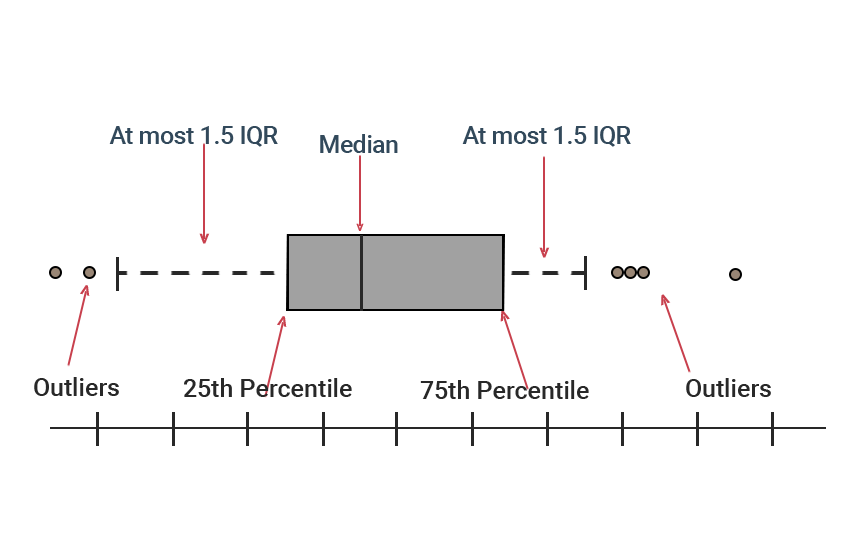What is the information provided by a Box Plot?

Box Plot will provide the following information

• Median (Q2/ 50th Percentile): The Middlemost value
• First Quartile (Q1/25th Percentile): The middle number between the smallest number and median of the data
• Third Quartile (Q3/75th Percentile): The middle number between the highest number and median of the data
• Whisker: A-line extending vertically from Box. Hence, “Box Plot” is also called “Whisker Plot". Whisker represents a spread of 25% of the data (lower & upper whiskers)
• Outliers: Any data not included between the whiskers is plotted as an outlier with a dot
• Inter Quartile Range (IQR): This is a measure of the difference between 75th and 25th percentiles simply, IQR = Q3 – Q1
• Minimum: (Q1-1.5*IQR)
• Maximum: (Q3+1.5*IQR)

### Box Plot on Normal Distribution:

Let us try and understand the Box Plot on a normal distribution and the probability density function.

Box Plot on Normal Distribution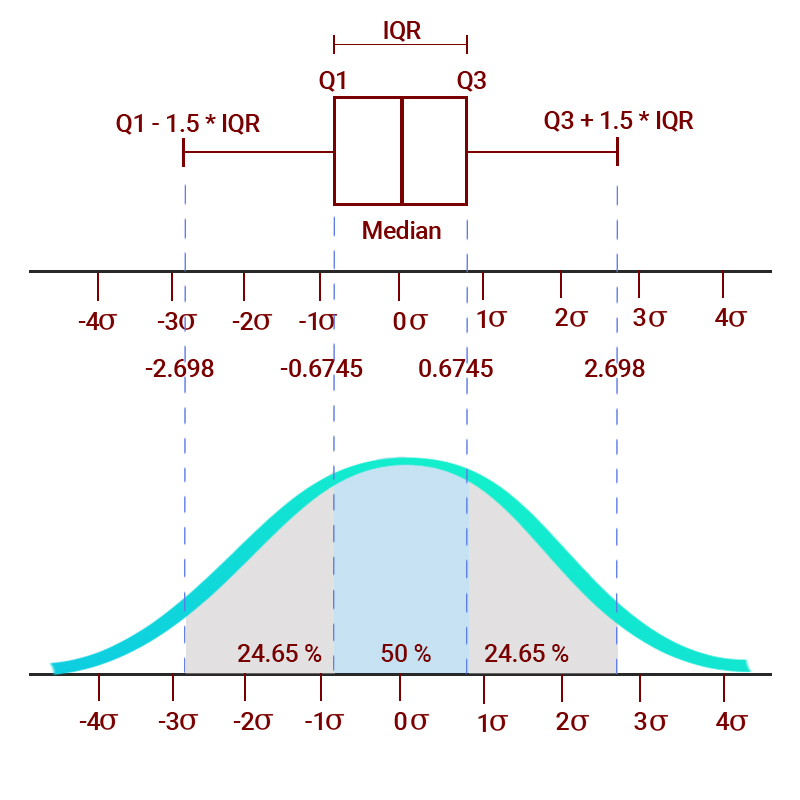What is a normal distribution?

The normal distribution is explained with a 68 - 95 - 99.7 rule

Points to be considered here are:

• 68% of the data is within 1 standard deviation (σ) and of the mean (μ)
• 95% of the data is within 2 standard deviations (σ) and of the mean (μ)
• 99.7% of the data is with 3 standard deviations (σ) and of the mean (μ)
• 7% of Outliers

Note: Boxplot can be constructed on non-normal data also

Let us now understand more about Boxplot

The basic format of the box plot is to use a box to convey the middle 50% of the data. This region is called as InterQuartile Range - IQR.

Several variations on the traditional “Box Plot” exist. The most common among them are Variable Width Box Plot and Notched Box Plot.

• Variable Width Box Plot: It illustrates the size of each group of data by making the width proportional to the size of the group
• Notched Box Plot: It applies a “notch” or narrows at the median of the box. The width of notches is proportional to the IQR of the sample and inversely proportional to the square root of the size of the sample

### Math Behind Box Plot:

Consider a sample of 10 data points

11, 16, 12, 17, 14, 12, 16, 17, 13, 20

• Order the data from smallest to largest
11, 12, 12, 13, 14, 16, 16, 17, 17, 20
• Find the Median

Median is the middlemost value for odd numbers of sample data, or it is the average of two middle numbers for even numbers of sample data i.e. 11, 12, 12, 13, 14
Q1 = 12

The third quartile if the median of the data points to the right of the median.i.e. 16, 16, 17, 17, 20
Q3 = 17

• Complete the five-number summary by finding the minimum and maximum value in the dataset
• The minimum is the smallest data point, which is 11
• The maximum is the largest data point, which is 20
• The five-number summary is 11, 12, 15, 17, 20

## CONSTRUCTION OF A BOX PLOT USING THE ABOVE DATA

• Mark an axis that fits the above five-number summary

BOX PLOT AXIS• Draw a box from Q1 to Q3 with a vertical line through a median

Q1 = BOX PLOT DEMARCATING MEDIAN AND QUARTILES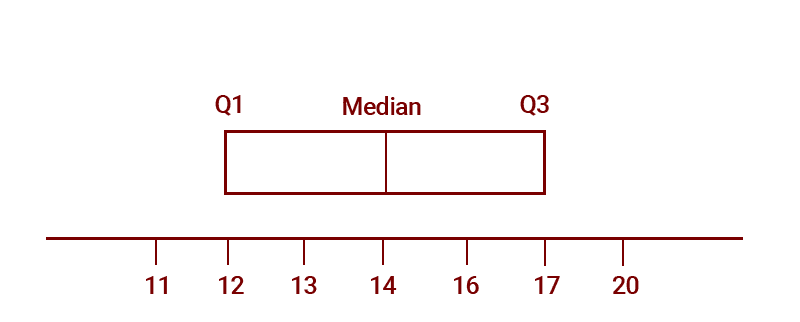• Draw a whisker from Q1 to the min and from Q3 to max
Min = 11 and Max = 20
Q1 = BOX PLOT DEMARCATING WHISKERS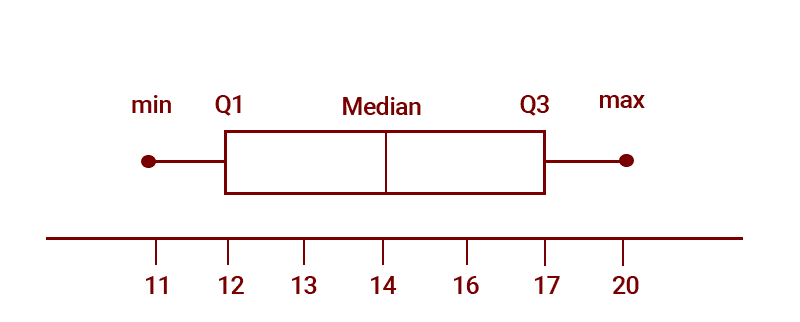## Interpreting the Quartiles

The five-number summary divides the data into sections where each section contain 25% of the data in that set.
BOX PLOT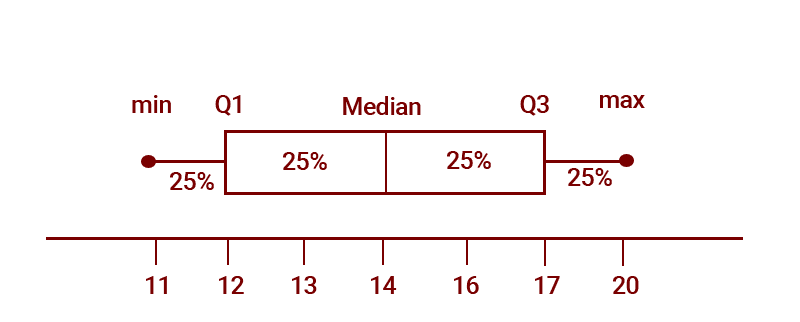Since Q1=12, about 25% of the data is lower than 29 and about 75% is above 29.

Outliners:
If the data happens to be normally distributed, then IQR = 1.35 σ where σ is a standard deviation.

Outliers = 1.5 * IQR times more above the third quartile or below the first quartile.

Note that outliers are not necessarily always “bad”, they may be the most important and most informative part of the dataset. They should not be removed without properly verifying. Outliers are very important and require special treatment; they may be the key understudy or they may be the result of human errors.

Best Data Science Course in Bangalore

With the help of the Box Plot, I tried to derive insights for 10 different Universities. However, I will try to explain using one feature (Salaries) from the University data. (Please note for this article I have masked data points as it contains some sensitive information).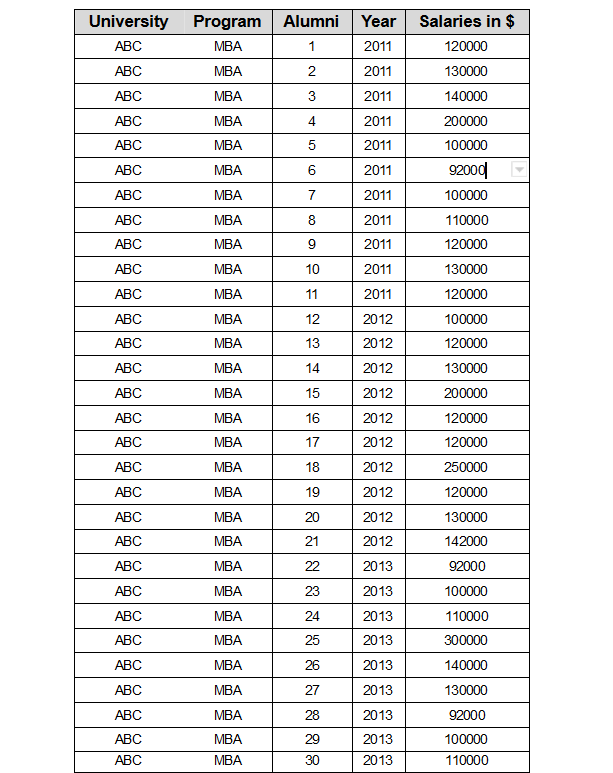I have used Data Science tools like R and Python to come up with these insights.

## R programming:

• “file.choose()”: The argument to load the dataset using GUI
• “attach()”: Function defines the content of the object. Used to call the column name directly without referring to the table name in R
• “names()”: Function to show the column names

I have created a Box Plot to identify some of the outliers in the data. If I remove them the average salary will get affected.

BOX PLOT CODE IN R LANGUAGE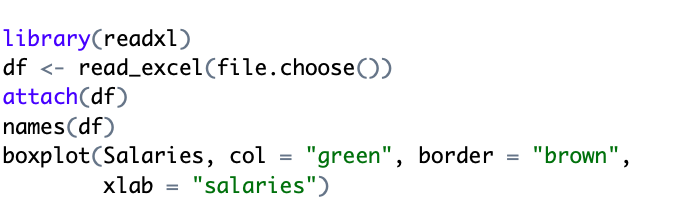The output of the box plot will look like the below image which shows that there are some outliers, which are influencing the mean calculation. BOX PLOT CONTENT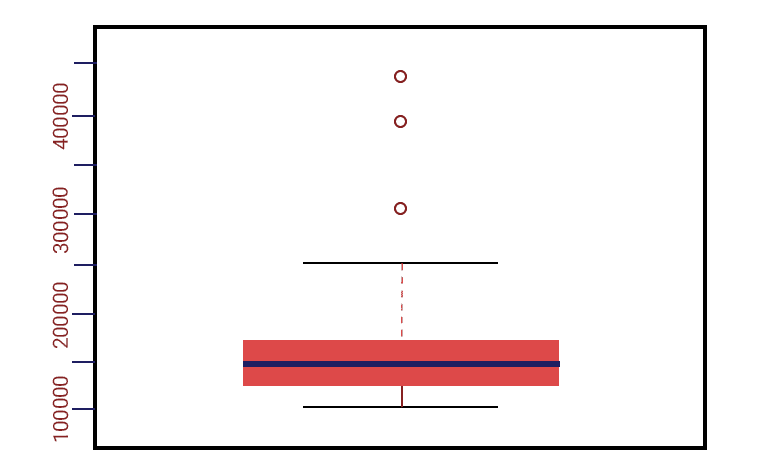Calculation of IQR: I need Q1 and Q3 for which an inbuilt function quantile() is used to calculate percentiles 0.25 and 0.75 respectively.USING QUANTILE FUNCTIONOutliers = (Q1 - 1.5 * IQR) and (Q3 + 1.5 * IQR).

Calculations of Outliners:CODE FOR OUTLIERS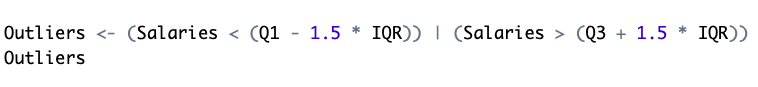Now I have outlier’s data which I am appending to the original file

APPENDING OUTLIERS DATA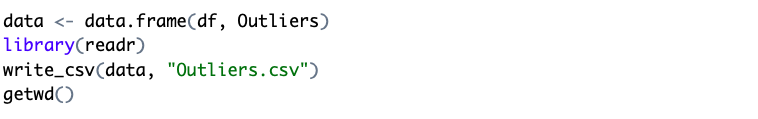I will share this final analysis report loaded into the file Outliers.csv with my friend so that he can now make a wise decision of choosing the right university.

I can achieve the same using Python Programming as well

Python Programming

• Import the required libraries and import the dataset.

IMPORTING PYTHON LIBRARIES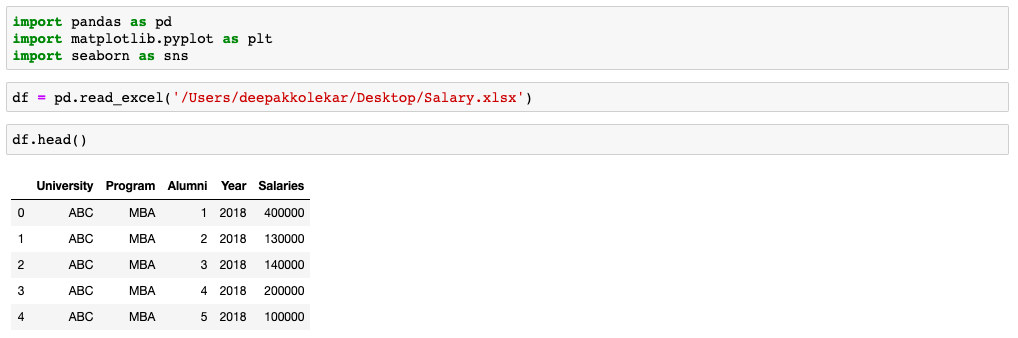IMPORTING PYTHON DATASETS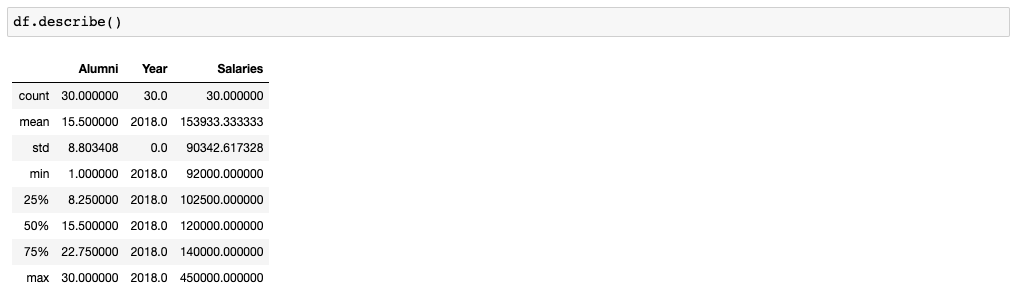• In Python, I used the seaborn library for the boxplot function

PYTHON CODE FOR BOX PLOT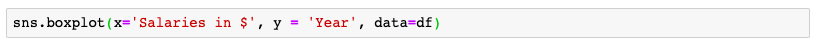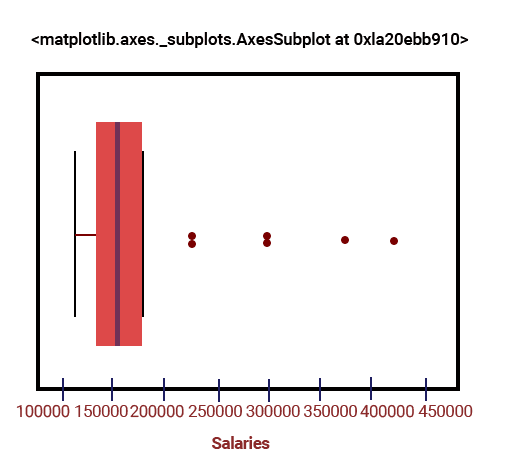Used swarmplot() to get a better representation of the distribution of the data. However, if the data is large then this representation would not be an ideal one.SWARMPLOT FUNCTION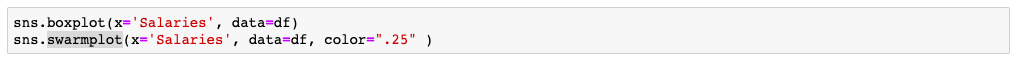The output shows the distribution of data points along with the boxplotBOX PLOT OUTPUT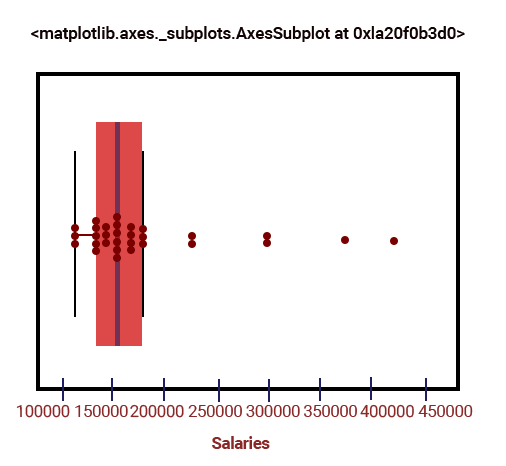We can see that there are some outliers however, we need to know what those outliers are

In order to calculate outliers mathematically, we need to come up with IQR (Inter Quartile Range) which is IQR = Q1 (Quartile 1) – Q3 (Quartile 3) i.e. 25th and 75th percentile.

• In python we have a function quantile() to calculate percentiles, using Q1 and Q3 it can be calculatedIMPORTING PYTHON LIBRARIESQUANTILE FUNCTIONWith IQR I calculate outliers using the formula (Q1 – 1.5* IQR) and (Q3 + 1.5*IQR). The results from the python code will return as Boolean for outliers, it will print either as True or False. CODE FOR OUTLIERS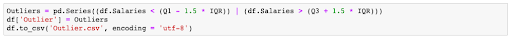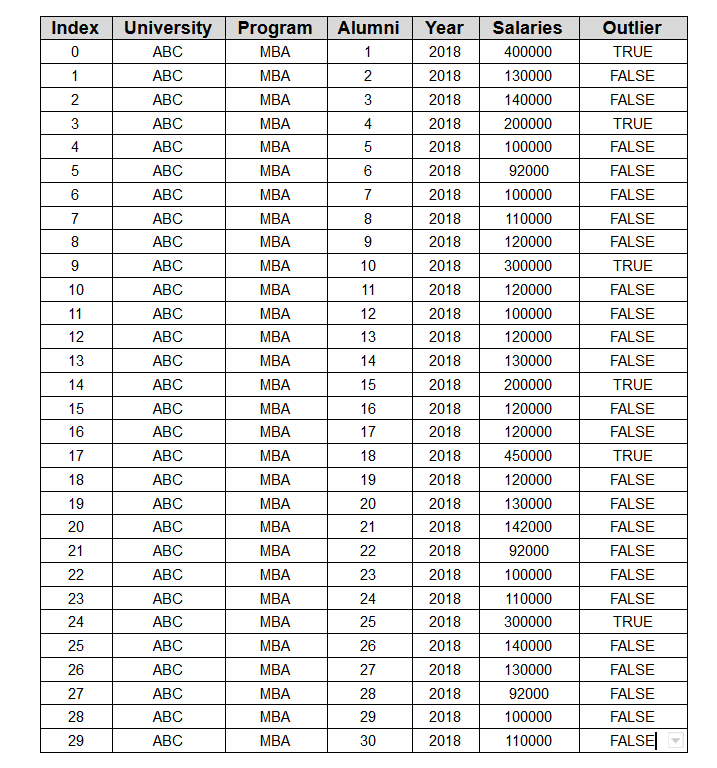SALARIES OF UNIVERSITY STUDENTS AFTER BOX PLOT ANALYSIS

Conclusion : Data science is all about sharing findings with audiences that might not be familiar with these undiscovered ideas. I was able to understand things from the analysis that I would not have otherwise. The outcomes of the statistical computations should guide my friend's decision-making.

I would warn my buddy not to be duped by the inflated salaries that certain universities are quoting.

Click here to learn Data Science Course, Data Science Course in Hyderabad, Data Science Course in Bangalore

### Data Analyst Courses in Other Locations